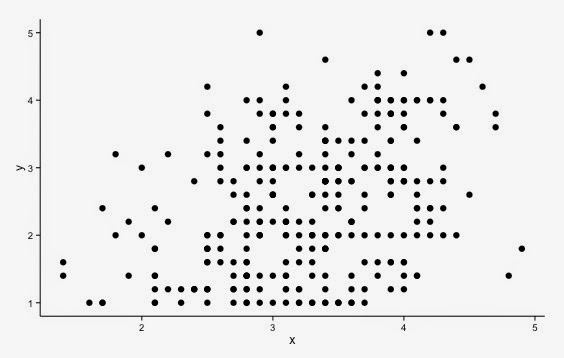## Posts

Showing posts from April, 2015

### Jittering in R (ggplot2)Jittering is the act of adding random noise to data in order to prevent overplotting in statistical graphs. Overplotting can occur when a continuous measurement is rounded to a convenient unit. This has the effect of making a continuous variable appear like a discrete ordinal variable.  For example, age is measured in years and body weight is measured in pounds or kilograms.  A scatter plot of weight versus age, which includes a sufficiently large sample of people will involve considerable overlap. Many individuals may be recorded as, 29 years old and weighing 70 kg, and there will be many markers plotted at the point (29, 70). The same is often true when plotting other individual difference metrics throughout psychology (e.g. personality) (Figure 1). Figure 1: Before Jittering - a significant positive correlation between x & y [r = .37]. To alleviate overplotting, it is possible to add a small amount of random noise to the data  (Figure 2) . The size of the noise is